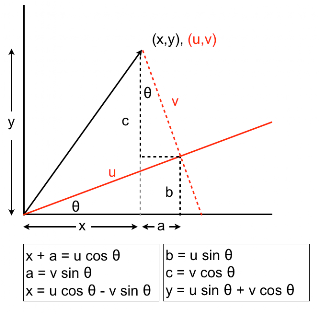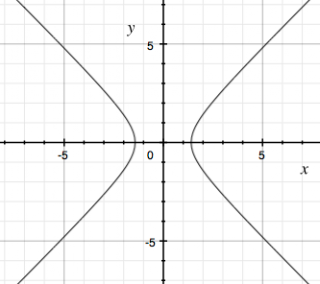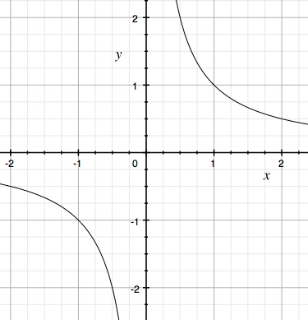Monday, June 27, 2011

Rotating a hyperbola: general case

Just to finish up quickly with the analysis of hyperbolas from the other day (here and here), following Stewart (pdf), we derived the following equations for x and y in terms of a coordinate system based on u and v (orthogonal, rotated through angle θ):

 x = u cosθ - v sinθy = u sinθ + v cosθ

The general formula for a quadratic is:

 Ax2 + Bxy + Cy2 + Dx + Ey + F = 0

When we transform to the new coordinates, we get:

 Ax2 = A[u2 cos2θ - 2uv sinθ cosθ + v2 sin2θ]Bxy = B[u2 cosθ sinθ + uv cos2θ - uv sin2θ - v2 sinθ cosθCy2 = C[u2 sin2θ + 2uv sinθ cosθ + v2 cos2θ

Gathering the terms in uv we obtain the coefficients:

 (C-A)(2sinθ cosθ) + B(cos2θ - sin2θ)

Remember the double angle formulas:

 sin(s+t) = sin s cos t + cos s sin tsin(2θ) = 2 sinθ cosθcos(s+t = cos s cos t - sin s sin tcos(2θ) = cos2θ - sin2θ

So we obtain:

 (C-A) sin(2θ) + B cos(2θ)

for the coefficients of xy. These must equal zero for all the xy terms to disappear. Thus:

 tan(2θ) = B / (C-A)

This approach runs into a problem if C = A, as it does for our example:

 xy = 1

But we can just invert the step at the end:

 cot(2θ) = (C-A)/B = 0

The cotangent is zero when the cosine is zero, e.g. for

 2θ = π/2

Thus, if

 θ = π/4

then all the xy terms vanish, as we found before.

Sunday, June 26, 2011

Mistakes

A few months ago I posted briefly about Sal Khan's academy (here, his link here). He has put up an extraordinary number of talks (1000?) where you see video of what he's "writing" on the screen and Sal talks you through explanations of various topics. It's mostly math, but also some biology, even finance. If you don't know about it, check it out.

There's a lot to like about this project. For starters, Sal knows his linear algebra and calculus, not to mention algebra. Also, it's free. Also, the lectures come as bite-sized chunks which is just right for the modern student with an attention span measured in single-digit minutes if not seconds. One negative aspect is that the system for projecting what he writes is pretty marginal---some kind of a pen would be a lot better.

But the reason I'm re-posting about this is I found a rather egregious error in one of the videos, and it reminded me of my own experience. The error is central to the example (in probability and statistics), in which he explains that "the standard deviation is just the average distance from the average," and proceeds to calculate just as you would if that were true. Of course, it's not true.

Why it's done the way it is, that's a whole 'nother topic.

But that's not my point. This error reminded me of my first teaching experience, long ago. I was a first-year graduate student, and I had barely noticed that I was scheduled to run a "recitation section" for a eukaryotic genetics class. I left my DNA samples on ice and ran to the first meeting of class. Unfortunately, the students expected me to actually explain stuff to them, like what was in the reading assignment on meiosis.

Hmm... meiosis.

I thought I remembered that. Unfortunately, I remembered wrong. Homologs segregate at division 1, not division 2. Oops.

Almost none of the students in my section turned up for week 2! In fact, the other TA accused me of screwing up on purpose, so she would have more students in her section. (Cindy, I'm sorry, I really am).

Anyway, I did learn my lesson. You must know the material when you walk in the door. And if you don't, be honest (and show up next time with the answer).

I think a similar thing happened to Sal. He has so many videos that he failed to prepare. As James Baker said in his biography: 'prior preparation prevents poor performance.' The five P's. A motto to live by.

Rotational transformation: geometrical viewA bit more about rotational transformations (see the previous post for the setup to this). We derived equations describing the coordinates of a point at x,y with respect to a new coordinate system in u,v that is rotated counter-clockwise by an angle θ:

 x = u cos(θ) - v sin(θ)y = u sin(θ) + v cos(θ)

We can also solve these equations for u and v. (It helps to know the answer). Notice that if we multiply the first equation by cos(θ) and the second one by sin(θ) and add, we'll end up with u by itself on the right-hand side:

 x cos(θ) = u cos2(θ) - v sin(θ) cos(θ)y sin(θ) = u sin2(θ) + v cos(θ) sin(θ)x cos(θ) + y sin(θ) = u cos2(θ) + u sin2(θ) x cos(θ) + y sin(θ) = u

Similarly, if we multiply the first equation by sin(θ) and the second one by cos(θ) and subtract the first from the second, we end up with v by itself:

 x sin(θ) = u sin(θ) cos(θ) - v sin2(θ)y cos(θ) = u sin(θ) cos(θ) + v cos2(θ)- x sin(θ) + y cos(&theta) = v cos2(θ) + v sin2(θ)- x sin(θ) + y cos(&theta) = v

I came up with geometric explanations for these relationships, the one for x and y in terms of u and v is at the top of the post, and below is the same drawing but explaining u and v in terms of x and y.A quicker way to the same place is to start by considering u and v to be the original coordinate system, and rotate clockwise through the angle θ. Then θ is negative, sin(θ) = -sin(-θ) and the cosine stays the same. We end up switching the signs of the sine terms, as we found.

Different views, same hyperbola

In the algebra study guide that my son is using (ALEKS) they set up a hyperbola in two "standard" forms as:

 (x-h)2/a2 - (y-k)2/b2 = 1(y-k)2/b2 - (x-h)2/a2 = 1

or more generally:

 (x-h)2/a2 - (y-k)2/b2 = +/- 1

Let's simplify our lives and consider an example centered at the origin:

 x2/a2 - y2/b2 = 1

Then they divide the world into those hyperbolas that open up and down versus those that open up left and right.

By looking at the equation, I think we can agree that if x2 equals 0 we've got a problem, since no value of y can satisfy the equation, and in fact x must not be less than 1. Hence, we can already predict that this system opens left and right, as the plot shows (a2 = b2 = 2):The ALEKS review goes on to explain that for this type of equation, the vertices of the hyperbola (points of closest approach to the origin at h,k) are equal to

 h +/- a, k

and the asymptotes have slopes equal to

 +/- b/a

This all works great. The problem I had was that the simplest parabola I can think of is:

 xy = 1

which doesn't fit the system.The answer to my confusion is that any parabola may be rotated around its origin in the xy-plane. In 2 selected orientations its equation will have only x2 and y2 terms (when the vertices are on the x or the y-axis), whereas in 2 other selected orientations it may have only xy terms (when the vertices are on y= +/- x. The rest of the time it contains both.

This is explained in an excerpt from Stewart's Calculus I found here.

Consider a point P in the plane with coordinates x,y and distance from the origin r. Now, let's establish a new coordinate system u,v which is rotated counter-clockwise by the angle θ. A vector from the origin through P is rotated an angle φ with respect to the u axis and φ + θ with respect to the x-axis. Here's a screenshot from the pdf:(Note, he uses capital X and Y for the second set of coordinates).

In the u,v system, the point P has coordinates

 u = r cos(φ)v = r sin(φ)

In the x,y system the coordinates are:

 x = r cos(φ + θ)y = r sin(φ + θ)

We remember (my post here) that:

 sin(s+t) = sin s cos t + cos s sin tcos(s+t) = cos s cos t - sin s sin t

Hence:

 x = r cos(φ) cos(θ) - r sin(φ) sin(θ) = u cos(θ) - v sin(θ)y = r sin(φ) cos(θ) + r cos(φ) sin(θ) = v cos(&theta) + u sin(θ) = u sin(&theta) + v cos(θ)

Now, consider the hyperbola:

 xy = 1

Rewrite this in terms of u,v and multiply, giving 4 terms

 + u cos(θ) u sin(θ)+ u cos(θ) v cos(θ)- u sin(θ) v sin(θ)- v sin(θ) v cos(θ)

If θ equals π/4, then

 cos(θ) = sin(θ) = 1/√2

The two middle terms drop out and leave us with:

 u2/2 - v2/2 = 1

If the middle terms don't cancel, we're left with a mixture including some fraction of
u2/2, v2/2, and u times v.

Note: we rotated the coordinate system counter-clockwise, which has the effect of rotating the plot clockwise, when the coordinate system is viewed in standard orientation.

Saturday, June 25, 2011

One more thing about 3x3 determinants

While we're on the topic of determinants (see yesterday's post), rather than pounding on arithmetic drills, the other thing I would show algebra students is that you can use any row or column to set up the computation. That can be useful if one choice contains zeros.

The standard approach uses the top row:

 a b cd e fg h i

And the determinant is:

 a(ei-hf) - b(di-fg) + c(dh-eg)

Where the sign of the second term is negative by the checkerboard rule:

 + - +- + -+ - +

Multiplying out we obtain:

 aei - afh - bdi + bfg + cdh - ceg

The 6 terms contain three components, each taken from a different row and column. For example, the components of bdi are from:

 b = (1,2)d = (2,1)i = (3,3)

The checkerboard rule makes the sign come out correctly.

If we're working with the top row or the middle column and so processing b x (di - fg) or d x (bi - ch), we'll need the minus sign; whereas if we're obtaining this term from i x (ae - bd) we already have a minus sign.

Let's try using the last row. We have:

 a b cd e fg h ig(bf - ce) - h(af - cd) + i(ae - bd)bfg - cdg - afh + cdh + aei - bdi

Compare with the first example to see that all the terms are present.

Can we do it by the diagonal? Try ceg:

 c(dh - eg) - e(ai - cg) + g(bf - ce)

Nope. Some terms are correct, but some are duplicates.

Friday, June 24, 2011

Cramer's rule calculation

We have algebra homework that involves using Cramer's rule to solve not only 2 x 2 but also 3 x 3 systems. It seems kind of silly since this method is overkill for 2 x 2, and would never be used for 4 x 4 or larger.

(Note on the wikipedia article, start about halfway down, where it says "Explicit formulas for small systems".)

Also, and this gets closer to the point, drilling by solving 3 x 3 matrices is not really about the rule, which is pretty simple. It's about making an easy problem harder by stuffing a lot of arithmetic into it. And to me, that is symptomatic of a big difficulty with math education as I'm encountering it through my son. Computers are much better at computing sums than humans. It's just silly to drill students on arithmetic. If you want to do something complicated, why not derive Cramer's rule?

So, I decided to write a solver for 3 x 3 systems in Python. I wouldn't say it's thoroughly debugged yet, so let me know if you run into a problem. With the example shown, I did get the same answer as this online calculator.

The first code segment contains the equations explicitly entered as an array. I'm sure you know how to modify it to read input from a file.

test.py

 import numpy as npimport Cramerdef test_Cramer(): L = [2, 3, 0, 5, 1, 1, 1, 3, 2,-1, 3, 7] A = np.array(L) A.shape = (3,4) result = Cramer.solve(A) if result: x,y,z = result print 'solution' print 'x =', x print 'y =', y print 'z =', z, '\n' Cramer.check(A,x,y,z)test_Cramer()

The output looks like this:

 > python test.py solve[[ 2 3 0 5] [ 1 1 1 3] [ 2 -1 3 7]] compute 3 x 3 det of[[ 2 3 0] [ 1 1 1] [ 2 -1 3]]D = 5 compute 3 x 3 det of[[ 5 3 0] [ 3 1 1] [ 7 -1 3]]Dx = 14 compute 3 x 3 det of[[2 5 0] [1 3 1] [2 7 3]]Dy = -1 compute 3 x 3 det of[[ 2 3 5] [ 1 1 3] [ 2 -1 7]]Dz = 2 solutionx = 2.8y = -0.2z = 0.4 checkrow 0 = [2 3 0 5]2.0*2.8 + 3.0*-0.2 + 0.0*0.4 = 5.0 row 1 = [1 1 1 3]1.0*2.8 + 1.0*-0.2 + 1.0*0.4 = 3.0 row 2 = [ 2 -1 3 7]2.0*2.8 + -1.0*-0.2 + 3.0*0.4 = 7.0

Cramer.py

 import numpy as npdef det2x2(A, v=False): if v: print 'compute 2 x 2 det of' if v: print A assert A.shape == (2,2) return A*A - A*Adef det3x3(A): print 'compute 3 x 3 det of' print A assert A.shape == (3,3) a,b,c = A c1 = a * det2x2(A[1:3,[1,2]]) c2 = b * det2x2(A[1:3,[0,2]]) c3 = c * det2x2(A[1:3,[0,1]]) return c1 - c2 + c3 def solve(A): print 'solve' print A, '\n' assert A.shape == (3,4) D = det3x3(A[:,:3]) print 'D = ', D, '\n' if D == 0: print 'no solution' return Dx = det3x3(A[:,[3,1,2]]) print 'Dx = ', Dx, '\n' Dy = det3x3(A[:,[0,3,2]]) print 'Dy = ', Dy, '\n' Dz = det3x3(A[:,[0,1,3]]) print 'Dz = ', Dz, '\n' return Dx*1.0/D, Dy*1.0/D, Dz*1.0/D def check(A,x,y,z): print 'check' for i,r in enumerate(A): print 'row', i, '=', r pL = list() for coeff,var in zip(r[:3],(x,y,z)): c = str(round(coeff,2)) v = str(round(var,2)) pL.append(c + '*' + v) print ' + '.join(pL), print ' =', r*x + r*y + r*z, '\n'